# 5-local-tensions

 Importance: Medium ✭✭
 Author(s): DeVos, Matt
 Subject: Graph Theory » Topological Graph Theory » » Coloring
 Keywords: coloring surface tension
 Prize: bottle of wine (DeVos)
 Posted by: mdevos on: June 22nd, 2007
Conjecture   There exists a fixed constant(probably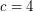suffices) so that every embedded (loopless) graph with edge-width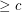has a 5-local-tension.

The edge-width of an embedded graph is the length of the shortest non-contractible cycle.

Definition   Letbe a directed graph, letbe an abelian group, and let. Define the height of a walkto be the sum ofon the forward edges ofminus the sum ofon the backward edges of(edges are counted according to multiplicity). We calla tension if the height of every closed walk is zero, and ifis an embedded graph, we calla local-tension if the height of every closed walk which forms a contractible curve is zero. If in addition,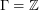andfor some, we say thatis a-tension or a-local-tension. If we reverse an edgeand replace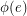by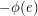, this preserves the properties of tension or local-tension. Accordingly, we say that an undirected graph (embedded graph)has a-tension (-local-tension) if some and thus every orientation of it admits such a map.
Proposition   A graph has a-tension if and only if it is-colorable.
Proof   To see the "if" direction, let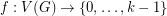be a coloring, orient the edges ofarbitrarily, and definingby the rule. It is straightforward to check thatis a-tension. For the "only if" direction, letbe a-tension. Now choose a pointand define the map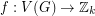by the rule that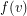is the height of some (and thus every) walk fromtomodulo. Again, it is straightforward to check that this defines a proper-coloring.

For graphs on orientable surfaces, local-tensions are dual to flows. More precisely, ifandare dual graphs embedded in an orientable surface, thenhas a-local-tension if and only ifhas a nowhere-zero-flow. On non-orientable surfaces, there is a duality between-local-tensions inand nowhere-zero-flows in a bidirected. Based on this duality we have a couple of conjectures. The first follows from Tutte's 5-flow conjecture, the second from Bouchet's 6-flow conjecture.

Conjecture  (Tutte)   Every loopless graph embedded in an orientable surface has a 5-local-tension.
Conjecture  (Bouchet)   Every loopless graph embedded in any surface has a 6-local-tension.

So although, graphs on surfaces may have high chromatic number, thanks to some partial results toward the above conjectures, we know that they always have small local-tensions. For orientable surfaces, there is a famous Conjecture of Grunbaum which is equivalent to the following.

Conjecture  (Grunbaum)   Ifis a simple loopless graph embedded in an orientable surface with edge-width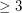, thenhas a 4-local-tension.

On non-orientable surfaces, it is known that there are graphs of arbitrarily high edge-width which do not admit 4-local-tensions (see [DGMVZ]). However, it remains open whether sufficiently high edge-width forces the existence of a 5-local-tension. Indeed, as suggested by the conjecture at the start of this page, it may be that edge-width at least 4 is enough. Edge-width 3 does not suffice since the embedding of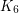in the projective plane does not admit a 5-local-tension.

## Bibliography

*[DGMVZ] M. DeVos, L. Goddyn, B. Mohar, D. Vertigan, and X. Zhu, Coloring-flow duality of embedded graphs. Trans. Amer. Math. Soc. 357 (2005), no. 10 MathSciNet

* indicates original appearance(s) of problem.